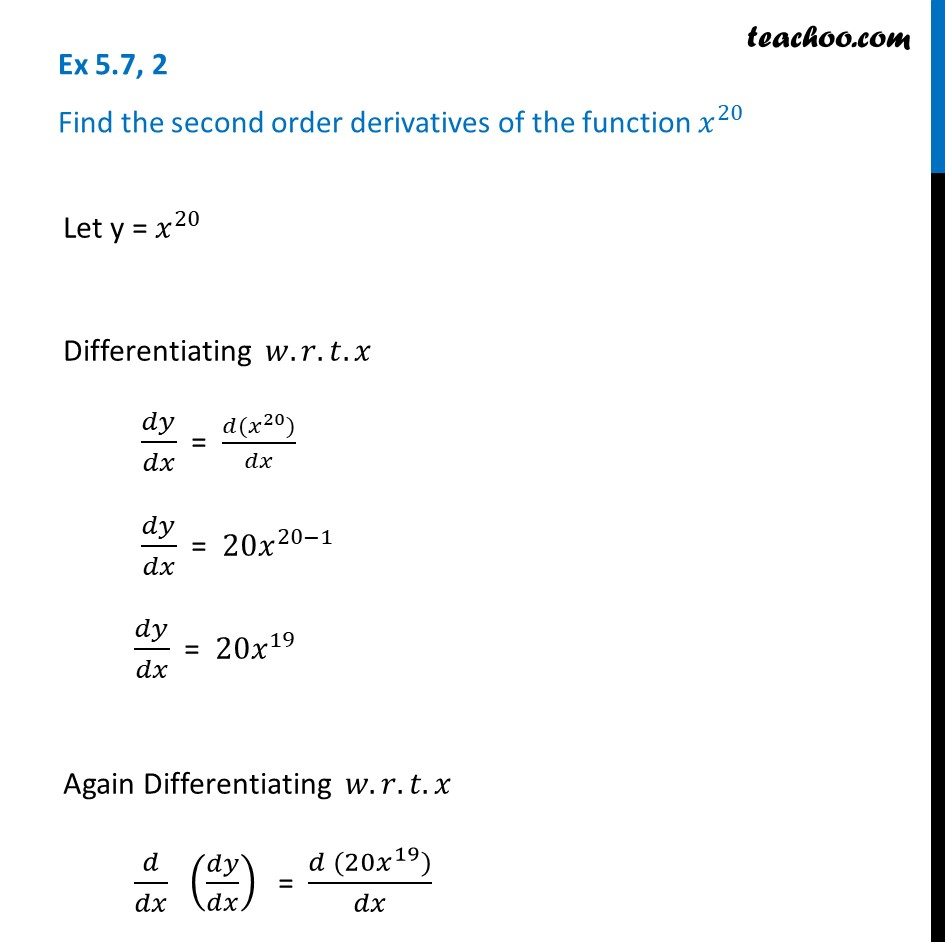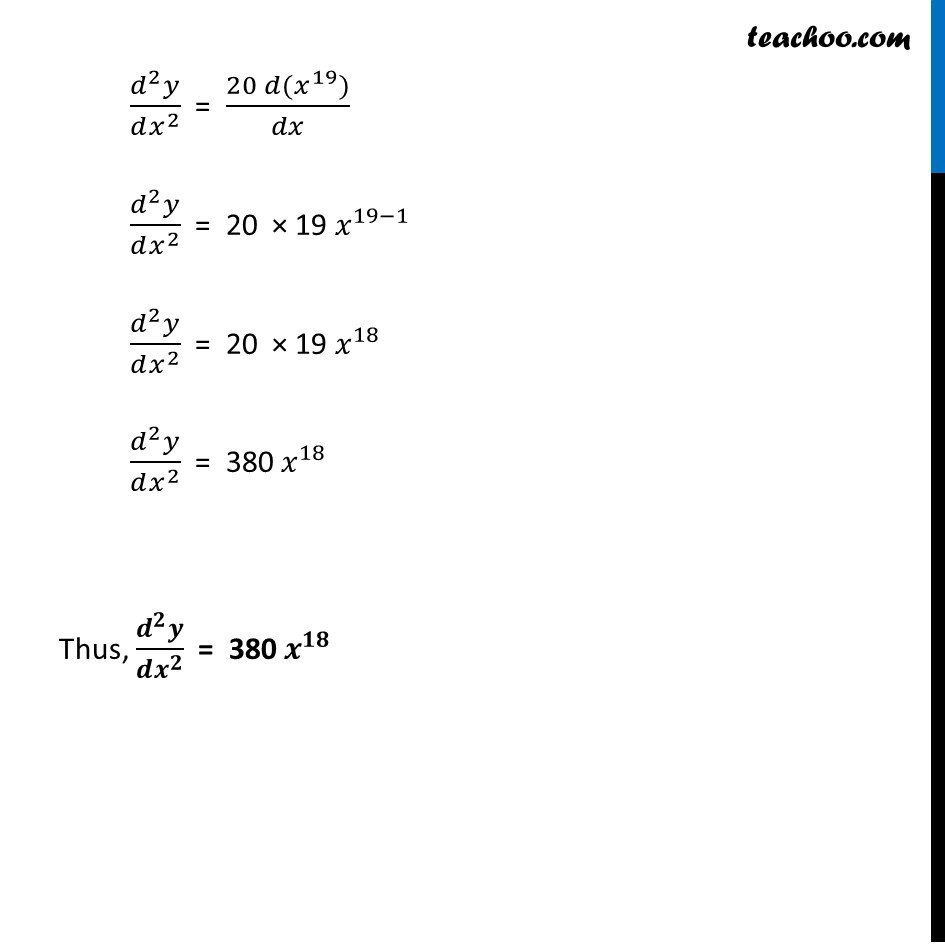Finding second order derivatives - Normal form

Chapter 5 Class 12 Continuity and Differentiability
Concept wiseIntroducing your new favourite teacher - Teachoo Black, at only ₹83 per month

### Transcript

Ex 5.7, 2 Find the second order derivatives of the function 𝑥^20 Let y = 𝑥^20 Differentiating 𝑤.𝑟.𝑡.𝑥 𝑑𝑦/𝑑𝑥 = (𝑑(𝑥^20))/𝑑𝑥 𝑑𝑦/𝑑𝑥 = 〖20𝑥〗^(20−1) 𝑑𝑦/𝑑𝑥 = 〖20𝑥〗^19 Again Differentiating 𝑤.𝑟.𝑡.𝑥 𝑑/𝑑𝑥 (𝑑𝑦/𝑑𝑥) = (𝑑 (〖20𝑥〗^19))/𝑑𝑥 (𝑑^2 𝑦)/(𝑑𝑥^2 ) = (20 𝑑(𝑥^19))/𝑑𝑥 (𝑑^2 𝑦)/(𝑑𝑥^2 ) = 20 × 19 𝑥^(19−1) (𝑑^2 𝑦)/(𝑑𝑥^2 ) = 20 × 19 𝑥^18 (𝑑^2 𝑦)/(𝑑𝑥^2 ) = 380 𝑥^18 Thus, (𝒅^𝟐 𝒚)/(𝒅𝒙^𝟐 ) = 380 𝒙^𝟏𝟖